top of page• # For a 2 x 2 matrix

Determinants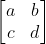• # For a 3 x 3 matrix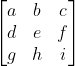# Consider a square formed by unit vectors i and j in x-y plane. Area of this square is 1 unit sq. A 2 x 2 matrix M, transforms this space and shifts i and j to# For a 3 x 3 matrix [aij] where i = 1,2,3 and j = 1,2,3; cofactor of element aij is:

Cofactor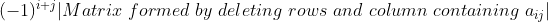# If A and B are two square matrix of same order such that [A][B] = I, then B is called inverse of matrix A.

Inverse# If a vector u is transformed to vector v by matrix M, inverse of M is a linear transformation matrix which brings vector v back to u.# Inverse of M is defined such that: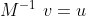# D) Both absolute value and sign remain unchanged

GATE previous questions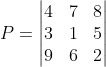# GATE 2014: Given that the determinant of matrix A is -12, the determinant of matrix B is, if A and B are as given below: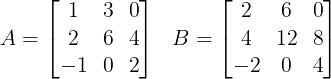# GATE 2018: For the following matrix A, find determinant of inverse of A.# Hence matrix A transforms a unit volume in space such that final volume is 4 unit. Inverse of A will reverse back this transformation and final volume will again become 1 unit. Hence;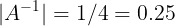# GATE 2015: For a matrix P as given below, the inverse of P is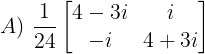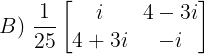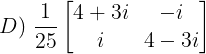# Matrix of Cofactors =# Adjoint of matrix P = Transpose of matrix C =# |P| = (4+3i)(4-3i) + i*i = 24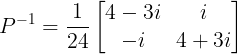bottom of page